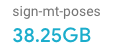# Library for viewing, augmenting, and handling .pose filessign-language-processing, updated 🕥 2023-03-28 10:53:09

# Pose Format

This repository aims to include a complete toolkit for working with poses. It includes a new file format with Python and Javascript readers and writers, in hope to make usage simple.

### The File Format

The format supports any type of poses, arbitrary number of people, and arbitrary number of frames (for videos).

The main idea is having a `header` with instructions on how many points exists, where, and how to connect them.

The binary spec can be found in docs/specs/v0.1.md.

### Python Usage

```bash pip install pose-format```

To load a `.pose` file, use the `PoseReader` class:

```python from pose_format.python.pose import Pose

buffer = open("file.pose", "rb").read() p = Pose.read(buffer) ````` By default, it uses NumPy for the data, but you can also use```torch`and`tensorflow` by writing:

```python from pose_format.python.pose import Pose from pose_format.python.torch import TorchPoseBody from pose_format.python.tensorflow.pose_body import TensorflowPoseBody

After creating a pose object that holds numpy data, it can also be converted to torch or tensorflow:

```python from pose_format.python.numpy import NumPyPoseBody

p.torch()

# return stored data as a tensorflow tensor

p.tensorflow() ```

### Common pose processing operations

Once poses are loaded, the library offers many ways to manipulate `Pose` objects.

#### Data normalization (skeleton scale)

To normalize all of the data to be in the same scale, we can normalize every pose by a constant feature of their body. For example, for people we can use the average span of their shoulders throughout the video to be a constant width. ```python p.normalize(p.header.normalization_info( p1=("pose_keypoints_2d", "RShoulder"), p2=("pose_keypoints_2d", "LShoulder") ))```

#### Data normalization (keypoint distribution)

Keypoint values can be standardized to have a mean of zero and unit variance: ```python p.normalize_distribution()```

The default behaviour is to compute a separate mean and standard deviation for each keypoint and each dimension (usually x and y). The `axis` argument can be used to customize this. For instance, to compute only two global means and standard deviations for the x and y dimension:

```python p.normalize_distribution(axis=(0, 1, 2))```

#### Data augmentation

```python p.augment2d(rotation_std=0.2, shear_std=0.2, scale_std=0.2)```

#### Data interpolation

To change the frame rate of a video, using data interpolation, use the `interpolate_fps` method which gets a new `fps` and a interpolation `kind`. ```python p.interpolate_fps(24, kind='cubic') p.interpolate_fps(24, kind='linear')```

### Visualization

Visualize an existing pose file:

```python from pose_format import Pose from pose_format.python.pose_visualizer import PoseVisualizer

v = PoseVisualizer(p)

v.save_video("example.mp4", v.draw()) ```

Draw pose on top of video:

```python v.save_video("example.mp4", v.draw_on_video("background_video_path.mp4"))```

Convert pose to gif to easily inspect the result in Colab:

```python

# in a Colab notebook

from IPython.display import Image

v.save_gif("test.gif", v.draw())

To load an OpenPose `directory`, use the `load_openpose_directory` utility:

directory = "/path/to/openpose/directory" p = load_openpose_directory(directory, fps=24, width=1000, height=1000) ```

### Testing

Use bazel to run tests ```sh cd pose_format bazel test ... --test_output=errors```

Alternatively, use a different testing framework to run tests, such as pytest. To run an individual test file: ```sh pytest pose_format/tensorflow/masked/tensor_test.py```

## Cite

```bibtex @misc{moryossef2021pose-format, title={pose-format: Library for viewing, augmenting, and handling .pose files}, author={Moryossef, Amit and M\"{u}ller, Mathias}, howpublished={\url{https://github.com/sign-language-processing/pose}}, year={2021} }```

## Issues

### 3D hand normalization with empty frames

opened on 2023-03-30 11:01:33 by J22Melody

I am trying the code from https://github.com/sign-language-processing/pose/pull/36.

When I input a .pose file where the first few frames are empty (=no right hand detected and all values are 0s), normalization output becomes all nans, where 0s are expected.

Since the pose data is a masked array, I use `pose.body.data.toflex()` to inspect the arrays and now I have to use `np.nan_to_num(pose.body.data)` as a workaround to convert the nans back to 0s.

@AmitMY maybe worth checking the 0-handing logic in the code?

### Can't save yuv420p videos when dimensions are odd

opened on 2023-03-28 14:23:14 by AmitMY

Current solution: ```py pose.header.dimensions.width += 1 if pose.header.dimensions.width % 2 == 1 else 0 pose.header.dimensions.height += 1 if pose.header.dimensions.height % 2 == 1 else 0```

Should just remove `yuv420p` if the frame is odd

### Allow storing poses with float16 instead of float32

opened on 2023-01-29 18:52:25 by AmitMY

We should allow storing `float16` poses, somehow, in v0.2, for storage efficiency### Add functions to quickly generate sample pose objects

opened on 2022-02-21 08:49:15 by bricksdont

Based on this discussion:

https://github.com/AI4Bharat/OpenHands/issues/29#issuecomment-1046607215

I think there is a need for code to quickly generate pose objects, with the Posebody type as a variable argument. I can take the code for this from unit tests, e.g. https://github.com/AmitMY/pose-format/blob/master/pose_format/pose_test.py#L159

This is what I had in mind:

```python random_pose_object = pose_format.Pose.random(pose_type="openpose_137", body_tpe="numpy", num_frames=10)```

## Releases

### v0.1.2 2022-09-08 21:15:07

Adds GIFs, and a very fast visualizer

v0.1.1

### v0.1.0 2022-07-27 14:05:59

v0.1.0 makes `pose-format` a lot slimmer by default, by making some dependencies optional.